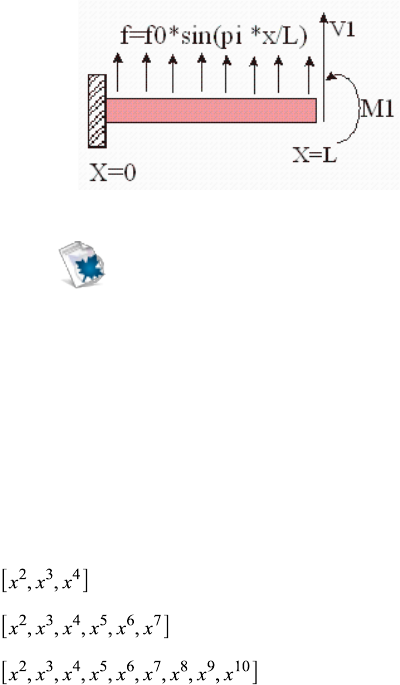Study Guides (390,000)
US (220,000)
TAMU (3,000)
AERO (10)
Final

# AERO 306 Lecture 16: 7_convergence_discontinuityExam

Department
Aerospace Engineering
Course Code
AERO 306
Professor
John Whitcomb
Study Guide
Final

This preview shows page 1. to view the full 4 pages of the document.C:\Users\cloudconvert\server\files\119\172\7\9e2d55cb-4bb6-4e18-8530-
527e8e2ab3f3\6677c020878463eb685f1d95472f06.docx p. 1 of 4
Convergence Behavior for Beam
This section summarizes the results from the following file.
beamConvergence.
mw
V1=10, M1=-400, L= 20, f0=5
This configuration was discussed earlier. This time we will consider the convergence behavior a
bit more seriously. This time we will consider polynomial approximations going up to a 9-term
solution.
Virtual work was used to obtain approximate solutions for the following problem. Three
approximations are used. Here are the interpolation functions.
So that we know the errors, the exact solution was also obtained by solving the fourth order
differential equation. This example demonstrates that a solution that appears to be exact for
displacements can actually have significant errors show up when three derivatives are taken to
calculate shear. It also demonstrates that if you take enough terms, the errors are very small.